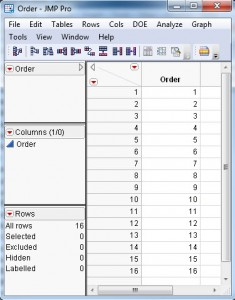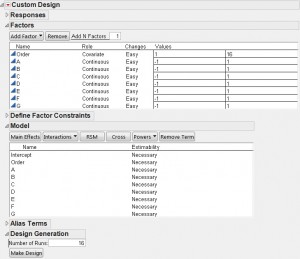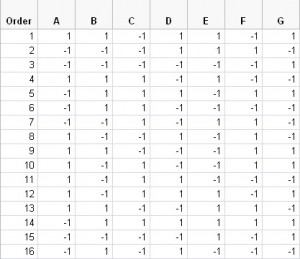How to create an experiment design that is robust to a linear time trend in the response

It is fairly common that experiments run in a time sequence experience linear drift in the response over the course of the experiment. If you randomize the order of the runs, then the effect of this drift will not tend to bias the estimated factor effects, but it will increase the variance of those effects.

Is there a better choice than random run order?

I would usually advise investigators to randomize the order of their runs or at least randomize the order within homogeneous groups of runs (blocks). However, if there is reason to suspect a strongly linear trend in the response over time independent of the factor changes, then there is a better way to proceed.

What is this better way?

In a previous post, I described how to add covariate factors to an experiment. In this case, one could consider time (or run order) to be a covariate factor. To make a design robust to trend, start by creating a data table that has one column. You could call it "Order." To make this example specific, I will create a 16-run experiment. So my data table for the covariate factor, Order, has 16 rows with the numbers one through 16 in order. I save my table and call it Order.jmp.Next, I open the Custom Designer and add Order as a covariate factor. For the example, I also add seven continuous factors and call them A through G. Figure 1 shows the custom design interface after entering the factors. The assumed model contains the main effects of all the factors.I want the most efficient design possible, so I go to the red triangle menu and choose the Number of Starts item. This choice controls the number of times the designer starts from a random starting design to try to find the best possible design. I choose 100,000 random starts, which took 33 seconds on my laptop.

Figure 2 shows the correlation color map of the design I created. The factors A through G are orthogonal to each other. Order is orthogonal to A, B, E and F. It is correlated with C, D and G, but the correlation is only 0.0271. These correlations lead to a variance inflation of less than 0.1% for the associated main effects.Table 2 shows the run order and factor settings for each run.

Bottom Line

It is not always possible to make the run order so nearly orthogonal to the factor effects as in this example. However, even if there are more substantial correlations between the Run Order column and other columns in the design, including Run Order as a factor in the analysis of the data accounts for any linear trend effect and allows for the precise estimation of the other factor effects.Labels
Article Tags